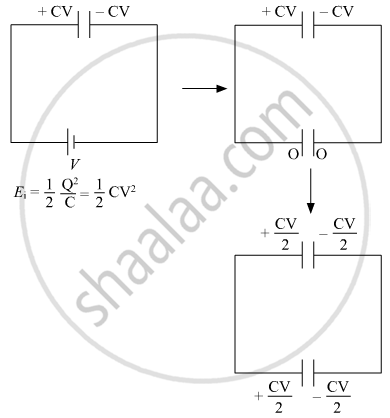# Solve the Following Question. a Parallel Plate Capacitor is Charged by a Battery to a Potential Difference V. It is Disconnected from the Battery and Then Connected to Another Uncharged Capacitor - Physics

Numerical

Solve the following question.
A parallel plate capacitor is charged by a battery to a potential difference V. It is disconnected from the battery and then connected to another uncharged capacitor of the same capacitance. Calculate the ratio of the energy stored in the combination to the initial energy on the single capacitor.

#### SolutionE_f = 1/2 ((CV)/2)^2/C + 1/2 ((CV)/2)^2/C

= (C^2V^2)/(4C)

E_f = 1/4 CV^2

E_f/E_i = (1/4 CV^2)/(1/2 CV^2) = 1/2

Concept: The Parallel Plate Capacitor
Is there an error in this question or solution?
2018-2019 (March) 55/1/3

Share